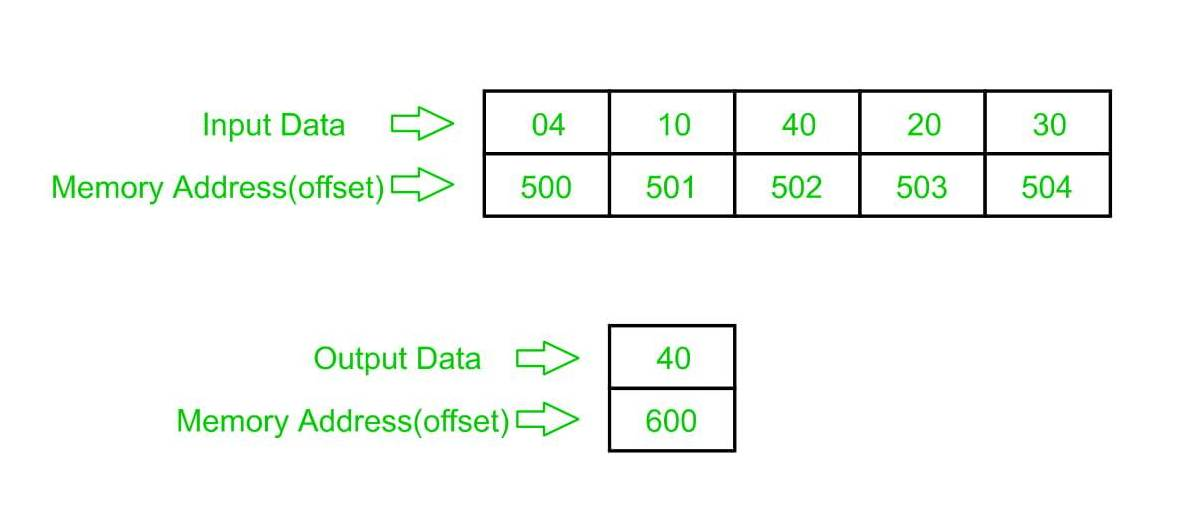# 8086 program to determine largest number in an array of n numbers

• Difficulty Level : Expert
• Last Updated : 22 May, 2018

Problem – Write a program in 8086 microprocessor to find out the largest among 8-bit n numbers, where size “n” is stored at memory address 2000 : 500 and the numbers are stored from memory address 2000 : 501 and store the result (largest number) into memory address 2000 : 600.

Example –Algorithm –

1. Load data from offset 500 to register CL and set register CH to 00 (for count).
2. Load first number(value) from next offset (i.e 501) to register AL and decrease count by 1.
3. Now compare value of register AL from data(value) at next offset, if that data is greater than value of register AL then update value of register AL to that data else no change, and increase offset value for next comparison and decrease count by 1 and continue this till count (value of register CX) becomes 0.
4. Store the result (value of register AL ) to memory address 2000 : 600.

Program –

400MOV SI, 500SI<-500
403MOV CL, [SI]CL<-[SI]
405MOV CH, 00CH<-00
407INC SISI<-SI+1
408MOV AL, [SI]AL<-[SI]
40CINC SISI<-SI+1
40DCMP AL, [SI]AL-[SI]
411MOV AL, [SI]AL<-[SI]
413INC SISI<-SI+1
416MOV , ALAL->
41AHLTEND

Explanation –

1. MOV SI, 500 : set the value of SI to 500
2. MOV CL, [SI] : load data from offset SI to register CL
3. MOV CH, 00 : set value of register CH to 00
4. INC SI : increase value of SI by 1.
5. MOV AL, [SI] : load value from offset SI to register AL
6. DEC CL : decrease value of register CL by 1
7. INC SI : increase value of SI by 1
8. CMP AL, [SI] : compares value of register AL and [SI] (AL-[SI])